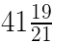Newbie

# A building is in the form of a cylinder surmounted by a hemisphere valted dome and contains 41 19/21 m cube of air. If the internal diameter of dome is equal to its total height above the floor, find the height of the building.

• 0

This question is Based on Mensuration Chapter of M.L Aggarwal book for ICSE BOARD for class 10.
A building is in the form of a cylinder surmounted by a hemisphere valted dome and contains air. If the internal diameter of dome is equal to its total height above the floor, find the height of the building.
This is the Question Number 17, Exercise 17.4 of M.L Aggarwal.

Share

1.Let the radius of the dome be r.

Internal diameter = 2r

Given internal diameter is equal to total height.

Total height of the building = 2r

Height of the hemispherical area = r

So height of cylindrical area, h = 2r-r = r

Volume of the building = Volume of cylindrical area + volume of hemispherical area

= r2h + (2/3)r3

= r3+ (2/3)r3 [∵h = r]

= r(1+2/3)

= r(3+2)/3

= (5/3)r3

Given Volume of the building == 880/21

(5/3)r3= 880/21

(5/3)×(22/7)×r3= 880/21

r= 880×3×7/(5×22×21)

r= 880/110

r= 8

Taking cube root

r = 2 m

Height of the building = 2r = 2×2 = 4m

Hence the height of the building is 4m.

• 1